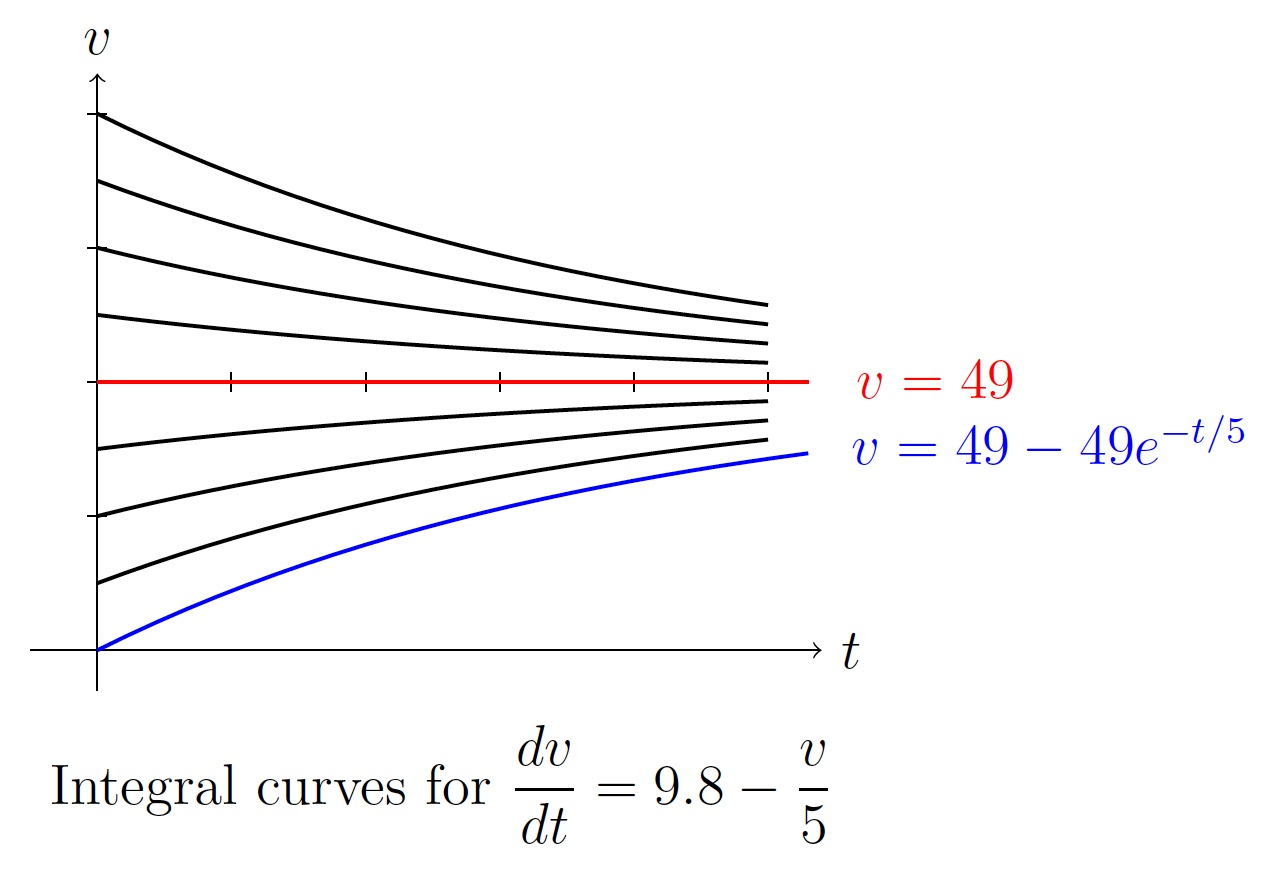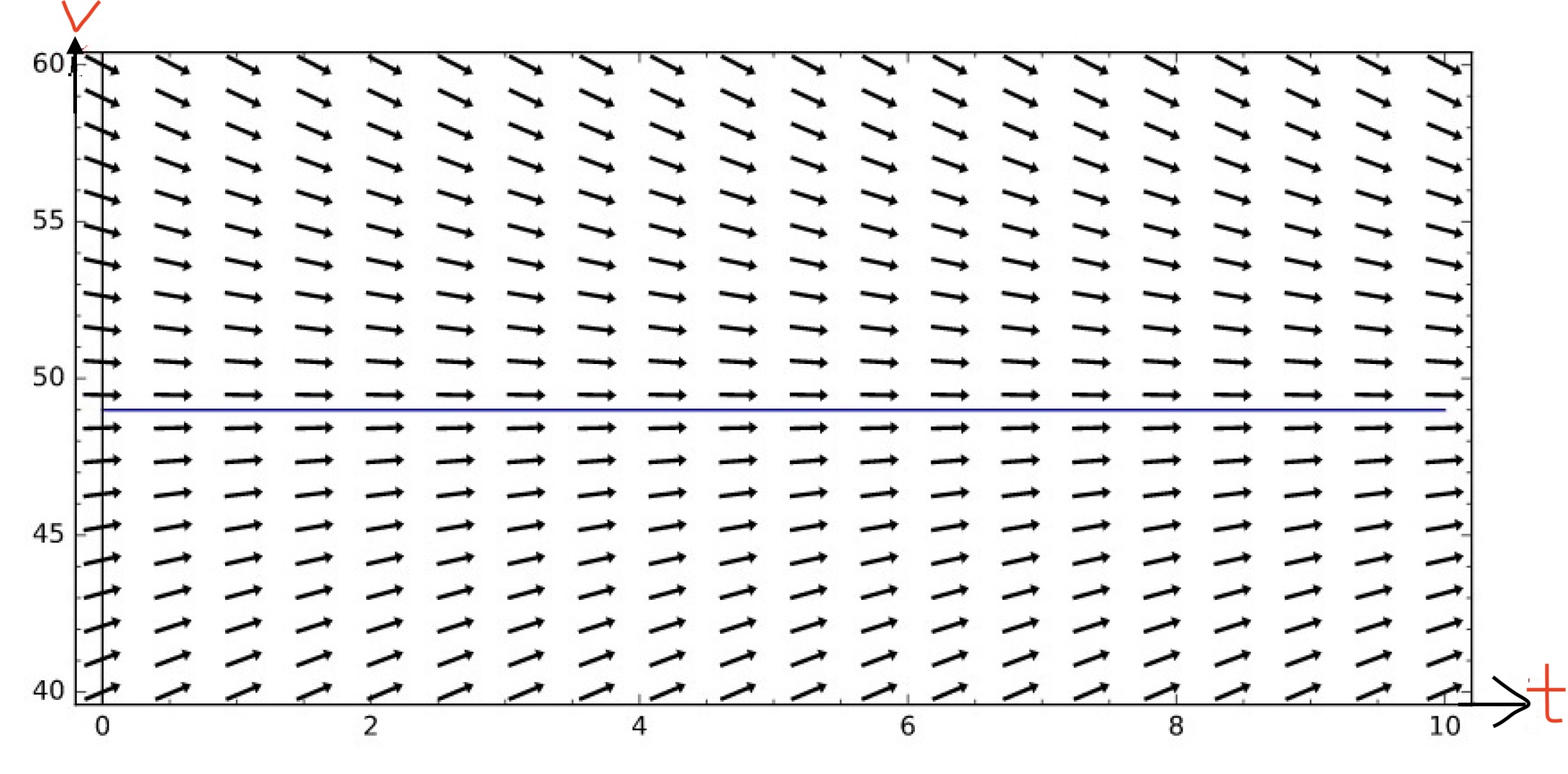DiffEq Home

## Terminology and Examples

A differential equation is an equation containing a function and its derivatives.

Example.

1. $$\displaystyle\frac{dy}{dx}=y\sin x$$

2. $$\displaystyle\frac{dv}{dt}=g-\frac{\gamma}{m}v$$ (motion of a falling object)

3. $$\displaystyle\frac{d^2\theta}{dt^2}+\frac{g\sin \theta}{L}=0$$ (motion of a pendulum)

4. $$\displaystyle\frac{\partial u}{\partial t}=k\frac{\partial^2u}{\partial x^2}$$ (one-dimensional heat equation)

Since derivative of a function means rate of change of the function, many physical phenomenon can be expressed or modelled as differential equations.

Motion of a falling object:
Suppose an object of mass $$m$$ is falling under the gravitational force. Suppose $$v$$ is its velocity at time $$t$$. So its acceleration is $$\displaystyle\frac{dv}{dt}$$. If $$F$$ is the force exerted on the object at time $$t$$, by Newton's second law of motion we have \begin{align*} F=m\displaystyle\frac{dv}{dt} && (1) \end{align*} Note that two external forces are acting on the object:

1. $$mg$$, the weight of the object ($$g$$ is the gravitational acceleration which is $$9.8\, m/s^2$$)

2. $$D$$, the drag or air resistance. It is modelled to be proportional to $$v$$. So $$D=\gamma v$$ where $$\gamma$$ is the drag coefficient which depends on the shape and the velocity of the object and the density of the air.

Thus $$F=mg-\gamma v$$. From (1), we have $\begin{equation*} m\displaystyle\frac{dv}{dt}=mg-\gamma v \end{equation*}$ Dividing both sides by $$m$$, we get \begin{align*} \displaystyle\frac{dv}{dt}=g-\frac{\gamma}{m} v&& (2) \end{align*}

A particular case:
Suppose a particle of mass $$5$$ kg is dropped. Suppose the drag coefficient is $$1\,kg/m$$. Then (2) becomes the following initial value problem (IVP): $\begin{equation}\label{eq2} \displaystyle\frac{dv}{dt}=9.8-\frac{v}{5},\; v(0)=0 \end{equation}$ Solve the above differential equation, i.e., find $$v$$ as a function of $$t$$. \begin{align*} \displaystyle\frac{dv}{dt}&=9.8-\frac{v}{5}=\frac{49-v}{5}\\ \displaystyle\frac{dv}{49-v}&=\frac{dt}{5}\\ \displaystyle\int\frac{dv}{v-49}&=\int\frac{dt}{-5}\\ \ln|v-49|&=-\frac{t}{5}+c,\text{ where $$c$$ is an integration constant} \\ |v-49|&=e^{c-t/5}=e^ce^{-t/5}\\ v&=49+ke^{-t/5},\text{ where }k=\pm e^c \end{align*}

Checking the solution: For $$v=49+ke^{-t/5}$$, $\displaystyle\frac{dv}{dt}=ke^{-t/5}\left(-\frac{1}{5}\right)=(v-49)\left(-\frac{1}{5}\right)=9.8-\frac{v}{5}.$ Note that for each value of $$k$$, $$v=49+ke^{-t/5}$$ satisfies the following differential equation \begin{align*} \displaystyle\frac{dv}{dt}=9.8-\frac{v}{5}&& (3) \end{align*} There are infinitely many solutions for differential equation (3). So $$v=49+ke^{-t/5}$$ is called the general solution of differential equation (3). Infinitely many solution curves corresponding to the general solution are called integral curves of the general solution. Assigning a particular value of $$k$$ in the general solution we get a particular solution to differential equation (3).We can find a particular value of $$k$$ from $$v=49+ke^{-t/5}$$ using the initial condition $$v(0)=0$$: $0=49+ke^0 \implies k=-49.$ So $$v=49-49e^{-t/5}$$ is the solution of the initial value differential equation (2).

End behavior of $$v$$:
Note that as $$t\to \infty$$, $$v=49+ke^{-t/5}\to 49$$.

Equilibrium Solution and Direction Fields:
An equilibrium solution is a particular solution to a differential equation that does not change with the independent variable, i.e., the derivative is zero. For the preceding problem, $$v=49$$ is an equilibrium solution. $\frac{dv}{dt}=9.8-\frac{v}{5}=0\implies v=49.$ Clearly $$\frac{dv}{dt}=9.8-\frac{v}{5} > 0$$ when $$v< 49$$ and $$\frac{dv}{dt}=9.8-\frac{v}{5} < 0$$ when $$v > 49$$.

In the $$t-v$$ graph, for a given value of $$v$$, we draw a vector with the slope $$\frac{dv}{dt}$$. For example, for $$v=49$$, we have $$\frac{dv}{dt}=0$$ and we get horizontal vectors on the line $$v=49$$. For $$v=45$$, we have $$\frac{dv}{dt}=0.8$$ and we get vectors with slope $$0.8$$ on the line $$v=45$$. This vector plotting on the $$tv$$-plane gives the direction field} or slope field of differential equation (3).Last edited